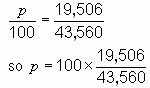SEARCH HOMEMath Central Quandaries & QueriesQuestion from Christine, a parent: Given that an acre is 43,560 sq/ft; If a piece of property is 19,506 sq/ft, What percentage of an acre is it?Hi Christine.

A percentage is just a fraction which is some number (the percentage) over 100. So the question is really this: what number divided by 100 is the same as 19,506 over 43,560 ?

Algebraically, we'd say:And using my calculator, 100 x 19,506 ÷ 43,560 = 44.78 (rounded off).

Cheers,
Stephen.Math Central is supported by the University of Regina and The Pacific Institute for the Mathematical Sciences.